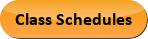Jun 10, 2023
2022-2023 College Catalog
 Select a Catalog 2023-2024 College Catalog 2022-2023 College Catalog [ARCHIVED CATALOG] 2021-2022 College Catalog [ARCHIVED CATALOG] 2020-2021 College Catalog [ARCHIVED CATALOG] 2019-2020 College Catalog [ARCHIVED CATALOG] *** DRAFT 2024-2025 College Catalog DRAFT *** [ARCHIVED CATALOG]
 HELP 2022-2023 College Catalog [ARCHIVED CATALOG] Print-Friendly Page (opens a new window)

# BUS 205 - Statistical Methods in Economics and Business [SUN# BUS 2201]

3 Credits, 3 Contact Hours
3 lecture periods 0 lab periods

Introduction to statistical concepts and methods of business. Includes statistics, data, and statistical thinking; methods for describing sets of data, probability, sampling distributions, inferences based on single sample and two samples; estimation with confidence intervals and tests of hypothesis; correlation and regression; time series; design of experiments; analysis of variance (ANOVA), and categorical data analysis.

Prerequisite(s): MAT 212  or MAT 220 .
Information: MAT 172  or MAT 173 may be accepted as the prerequisite if taken prior to Fall 2013. Basic Excel knowledge is required before enrolling in this course.
Gen-Ed: Meets AGEC - MATH; Meets CTE - M&S.Course Learning Outcomes
1. Determine the appropriate statistical test for a specific level of measurement.
2. Analyze the outcome of a t test.
3. Use a computer system in order to calculate demographics.

Performance Objectives:
1. Describe the different types of data collected and the fundamental elements of statistics.
2. Discuss descriptive statistics of data including measures of central tendency, variability and standard deviation, and relative standing.
3. Calculate probability.
4. Demonstrate sampling distributions and discuss the central limit theorem.
5. Explain confidence intervals and demonstrate statistical inferences based on a single population: tests of hypotheses.
6. Demonstrate statistical inferences based on two populations: confidence intervals and tests of hypotheses.
7. Explain correlation and regression.
8. Discuss time series data.
9. Discuss design of experiments and analysis of variance.
10. Use Excel or alternative statistical package to calculate statistics.

Outline:
1. Statistics, Data, and Statistical Thinking
1. Types of statistical applications in business
2. The role of statistics in managerial decision-making
3. Levels of measurement for data
2. Methods for Describing Sets of Data
1. Measures of central tendency
2. Variability and standard deviation
3. Z-scores
3. Probability
1. Series
2. Sequences
4. Sampling Distributions
1. Skewed distributions
2. Normal distributions
5. Inferences Based on a Single Sample: Estimation with Confidence Intervals and Tests of  Hypotheses
1. Large sample
2. Small sample
3. Z- tests
6. Inferences Based on Two Samples: Confidence Intervals and Tests of Hypotheses
1. Independent T-test
2. Paired/dependent T-test
7. Correlation and Regression
1. Correlation Pearson’s
2. Simple linear regression using the least squares approach
3. Multiple regression
8. Time Series
1. Descriptive analyses
2. Models
3. Trends
4. Forecasting
9. Design of Experiments and Analysis of Variance (ANOVA)
1. Single factor ANOVAs
2. Two factor ANOVAs
10. Categorical Data Analysis
1. One-way Chi-Square test
2. Pearson’s Chi-Square test